## Thursday, September 30, 2010

### 948

948 = 22 x 3 x 79.

948 is the number of times the digit 8 appears in the first 10,000 decimal digits of pi.

948 is a number n such that n3 is a sum of two successive primes: 9483 = 851971392 = 425985683 + 425985709.

The sum of the digits of 948 equals the 7 times the number of digits: 9 + 4 + 8 = 21 = 7 x 3.The USS Morton DD 948, a destroyer, was known as the "saltiest ship in the fleet."

## Wednesday, September 29, 2010

### 459

459 = 33 x 17.

459 is the smallest number n such that if you subtract it from its reverse, you get a natural number with the same digits as n: 954 - 459 = 495.

459 is the smallest number such that the product of its digits is 10 times their sum: 4 x 5 x 9 = 180 = 10 (4 + 5 + 9).

459 is 1224 in base 7 and 323 in base 12.Absolute zero on the Fahrenheit scale is about -459.67 degrees.

## Tuesday, September 28, 2010

### 573

573 = 3 x 191.

573 has the property that its square is the concatenation of two consecutive numbers: 5732 = 328329.

5733 = 188,132,517 = (188 - 132 + 517)3.

573 is 4243 in base 5 and 141 in base 22.573 Recha is an asteroid (minor planet) discovered in 1905.

## Monday, September 27, 2010

### 352

352 = 25 x 11.

352 has a unique representation as a sum of three squares: 352 = 82 + 122 + 122.

352 is the maximum number of volumes into which 11 spheres can divide space.

352 is the maximum number of regions into which 26 lines divide a plane.

352 is the only number consisting of three distinct prime digits such that the first and third equal the third raised to the power of the second (32 = 25).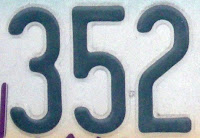352 is a telephone area code for a region around Gainesville, Florida.

## Friday, September 24, 2010

### 560

560 = 24 x 5 x 7.

560 has a unique representation as a sum of three squares: 560 = 42 + 122 + 202.

560 is the 14th tetrahedral number. 560 is also an octagonal number.

560 is 202202 in base 3 and 2332 in base 6.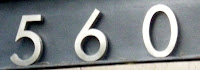560 is the number of different moves a bishop can make on a chessboard.

## Thursday, September 23, 2010

### 269

269 is a prime number.

269 has a representation as a sum of two squares: 269 = 102 + 132.

269 and 271 form a twin prime pair.

269 is the hypotenuse of a primitive Pythagorean triple: 2692 = 692 + 2602.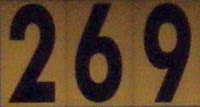The 269th day of a non-leap year is 26 September (26/9).

## Wednesday, September 22, 2010

### 736

736 = 25 x 23.

736 has a unique representation as a sum of three squares: 736 = 42 + 122 + 242.

736 has the representation 36 + 7.

736 is an octagonal number. It is also a centered heptagonal number.

736 is the smallest number such that n and 2n end with the same three digits.The Revox G36 (or 736 in Great Britain) was a mono/stereo reel-to-reel recording machine.

## Tuesday, September 21, 2010

### 128

128 = 27. It is the smallest number to be the product of seven prime factors.

128 is a power of 2, all of whose digits are themselves powers of 2.

128 is the number made by starting with 1 and doubling seven times: 1, 2, 4, 8, 16, 32, 64, 128.

128 = 9 + 11 + 13 + 15 + 17 + 19 + 21 + 23.

128 is 10000000 in base 2 (binary).

128 is the largest number that is not the sum of distinct squares.

128 has a representation as a sum of two squares: 128 = 82 + 82.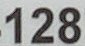Released in 1985, the Commodore 128 home computer was that last 8-bit machine commercially released by Commodore Business Machines.

Source: Phillips, R. 2004. Numbers: Facts, Figures and Fiction. Badsey Publications.

## Monday, September 20, 2010

### 331

331 is a prime number.

331 is a divisor of 313 - 1.

331 is good, happy, lucky, and cuban.The Best 331 Colleges by Robert Franek was published by Princeton Review in 2002.

## Friday, September 17, 2010

### 2233

2233 = 7 x 11 x 29.

2233 becomes a prime if any digit is deleted: 223 and 233 are primes.

2233 is a number such that each digit leaves the same nonzero remainder when each is divided into the number.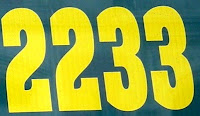A transit of the planet Mercury (as seen from Earth) will occur in the year 2233.

## Thursday, September 16, 2010

### 258

258 = 2 x 3 x 43.

258 is the minimum magic sum of a magic square using 16 consecutive primes.

258 is a value of n such that n(n + 9) is a palindrome: 258(267) = 68886.

258 is the sum of four consecutive primes: 258 = 59 + 61 + 67 + 71.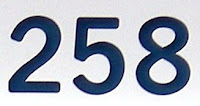258 Tyche is a relatively large asteroid discovered in 1886.

## Wednesday, September 15, 2010

### 242

242 = 2 x 112.

242 has a representation as a sum of two squares: 242 = 112 + 112.

242 is 22222 in base 3.

242 is the maximum number of regions into which 16 circles divide the plane.

All the prime factors and divisors of 242 are palindromic.

242 is the smallest number that is one larger than a prime (241) as well as being one less than the fifth power of another prime (243 = 35).A Belgian electronic music group in the 1980s went by the name of Front 242.

## Tuesday, September 14, 2010

### 829

829 is a prime number.

829 has a representation as a sum of two squares: 829 = 102 + 272.

827 and 829 form a twin prime pair.

829 is the hypotenuse of a primitive Pythagorean triple: 8292 = 5402 + 6292.

829 is a divisor of 59 - 1.

829 is the sum of three consecutive primes: 829 = 271 + 277 + 281.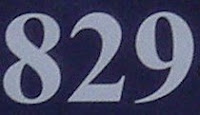829 is a telephone area code for the Dominican Republic.

Source: WolframAlpha

## Monday, September 13, 2010

### 520

520 = 23 x 5 x 13.

520 has two representations as a sum of two squares: 520 = 62 + 222 = 142 + 182.

520 is the number of ways to place two non-attacking kings on a six-by-six chessboard.

520 is 4040 in base 5 and 1010 in base 8.

520 is the sum of two successive primes: 520 = 257 + 263.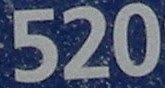NGC 520 appears to be a pair of colliding galaxies.

## Friday, September 10, 2010

### 36

36 = 22 x 32. It is a perfect square.

36 is 100100 in base 2 (binary). It is 44 in base 8.

36 has a unique representation as a sum of three squares: 36 = 22 + 42 + 42.

36 is the smallest number with four representations as a sum of two distinct primes: 36 = 5 + 31 = 7 + 29 = 13 + 23 = 17 + 19.

36 is the 8th triangular number (the sum of the first eight numbers). There are not many numbers that are both square and triangular. The first four are 1, 36, 1225, and 41616.

36 is the sum of the first three cubes: 13 + 23 + 33.

36 is the smallest number to have nine different factors: 1, 2, 3, 4, 6, 9, 12, 18, and 36.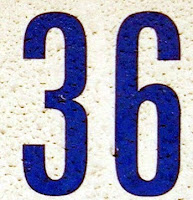William Shakespeare wrote 36 plays.

Source: Phillips, R. 1994 Numbers: Facts, Figures and Fiction. Cambridge University Press.

## Thursday, September 9, 2010

### 5008

5008 = 24 x 313.

5008 has a representation as a sum of two squares: 5008 = 482 + 522.

5008 is a divisor of 254 - 1.

5008 is 130013 in base 5.

5008 is the number of permutations of length 7 such that at least one absolute difference between consecutive elements has a distinct partner.The Peugeot 5008 is a compact family multipurpose vehicle introduced in 2009.

Source: WolframAlpha

## Wednesday, September 8, 2010

### 504

504 = 23 x 32 x 7.

504 = 24 x 21 = 42 x 12.

504 is 200200 in base 3 and 4004 in base 5.

504 is a refactorable number. The number of divisors of the number divides the number.Section 504 of the Rehabilitation Act of 1973 is a federal law designed to protect the rights of individuals with disabilities in programs and activities that receive federal financial assistance from the U.S. Department of Education.

## Tuesday, September 7, 2010

### 907

907 is a prime number.

907, 9907, 99907, 999907, and 9999907 are all primes.

907 is conjectured to be the largest prime that can be represented uniquely as the sum of three distinct positive squares: 907 = 32 + 132 + 272.

907 is 32023 in base 4.

Reversing 907 gives the prime 709.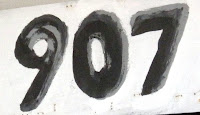907 is the telephone area code that covers that largest area of the United States, nearly all of the state of Alaska.

## Friday, September 3, 2010

### 84

84 = 22 x 3 x 7.

84 is tetrahedral number, the sum of the first seven triangular numbers: 84 = 1 + 3 + 6 + 10 + 15 + 21 + 28.

84 has a unique representation as a sum of three squares: 84 = 22 + 42 + 82.

84 is the smallest number with eight representations as a sum of two primes: 84 = 5 + 79 = 11 + 73 = 13 + 71 = 17 + 67 = 23 + 61 = 31 + 53 = 37 + 47 = 41 + 43.

84 is 77 in base 11 and 66 in base 13.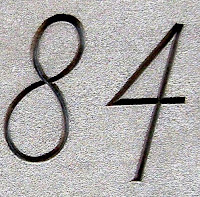84 Charing Cross Road is a book by Helene Hanff.

Source: Phillips, R. 1994. Numbers: Facts, Figures and Fiction. Cambridge University Press.